### Deriving the Momentum-Energy 4-Vector

The problem we have is how to take a time derivative if the time is the component of a 4-vector. We need some kind of scalar time to make sense of the equations we know and love. A well defined time, that does not need to be transformed, is the time in the rest frame of the particle. We call this the proper time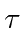. We will make use of it here, but later just try to rewrite our equations so that they are covariant in 4 dimensions.

The velocity 4-vector can be defined as.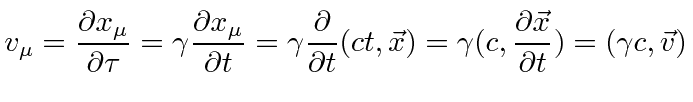So we see that the time-component of the usual velocity vector isand we have the velocity 4-vectorwhere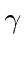is for the transformation from the rest frame to whatever frame we are definingin.

We can dot the velocity 4-vector into itself.This is certainly a scalar. It is an example of the problem that many 4D lengths'' are not very useful. That is, the magnitude of the velocity vector isno matter what the velocity is.

To be consistent with non-relativistic equations we will define the momentum.If we identify the time component as above,, we have the relationwhich looks similar to the rest energy equation but actually is true in any frame.

A crucial test of this derived'' 4-vector is whether it gives the right physics in the non-relativistic limit. We did have some choice to make when inserting the energy into the momentum 4-vector. Start with the energy equation from above.This is the correct non-relativistic limit. The total energy is, in the non-relativistic limit, the rest energy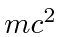plus the kinetic energy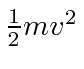. Normally, we ignore the rest energy as being unchangeable. It does change in nuclear interactions for example.

Jim Branson 2012-10-21